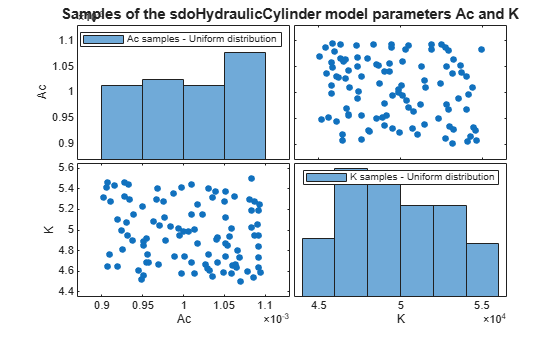Documentation

### This is machine translation

Mouseover text to see original. Click the button below to return to the English version of the page.

# sdo.scatterPlot

Scatter plot of samples

## Syntax

``sdo.scatterPlot(X,Y)``
``sdo.scatterPlot(X)``
``````[H,AX,BigAX,P,PAx] = sdo.scatterPlot(___)``````

## Description

example

````sdo.scatterPlot(X,Y)` creates a matrix of subaxes containing scatter plots of the columns of `X` against the columns of `Y`. If `X` is p-by-n and `Y` is p-by-m, then `sdo.scatterPlot` creates a matrix of n-by-m subaxes. `X` and `Y` must have the same number of rows.```

example

````sdo.scatterPlot(X)` is the same as `sdo.scatterPlot(X,X)`, except that the subaxes along the diagonal are replaced with histogram plots of the data in the corresponding column of `X`. For example, the subaxes along the diagonal in the ith column is replaced by `hist(X(:,i))`. ```

example

``````[H,AX,BigAX,P,PAx] = sdo.scatterPlot(___)``` returns the handles to the graphic objects. Use these handles to customize the scatter plot. For example, you can specify titles for the subaxes.```

## Examples

collapse all

Generally, you use the `sdo.scatterPlot(X,Y)` syntax with `X` specifying the samples and `Y` specifying the cost function value for each sample. Use the `sdo.evaluate` command to perform the cost function evaluation to generate `Y`. For this example, obtain 100 samples of the `Ac` and `K` parameters of the `sdoHydraulicCyclinder` model. Calculate the cost function as a function of `Ac` and `K`. Create a scatter plot to see the sample and cost function values.

Load the `sdoHydraulicCyclinder` model.

`load_system('sdoHydraulicCylinder');`

Generate 100 samples of the `Ac` and `K` parameters.

```p = sdo.getParameterFromModel('sdoHydraulicCylinder',{'Ac','K'}); ps = sdo.ParameterSpace(p); X = sdo.sample(ps,100);```

The first operation obtains the `Ac` and `K` parameters as a vector, `p`. The second operation creates an `sdo.ParameterSpace` object, `ps`, that specifies the probability distributions of the parameter samples. The third operation generates 100 samples of each parameter, returned as a `Table`, `X`.

Calculate the cost function value table.

```Ac_mean = mean(X{:,1}); K_mean = mean(X{:,2}); Y = table(X{:,1}/Ac_mean+X{:,2}/K_mean,'VariableNames',{'y'});```

Create a scatter plot of `X` and `Y`.

`sdo.scatterPlot(X,Y);`Sample the `Ac` and `K` parameters of the `sdoHydraulicCyclinder` model. Use a scatter plot to analyze the samples.

Load the `sdoHydraulicCyclinder` model.

`load_system('sdoHydraulicCylinder');`

Generate 100 samples of the `Ac` and `K` parameters.

```p = sdo.getParameterFromModel('sdoHydraulicCylinder',{'Ac','K'}); ps = sdo.ParameterSpace(p); X = sdo.sample(ps,100);```

The first operation obtains the `Ac` and `K` parameters as a vector, `p`. The second operation creates an `sdo.ParameterSpace` object, `ps`, that specifies the probability distributions of the parameter samples. The third operation generates 100 samples of each parameter, returned as a `Table`, `X`.

Create a scatter plot of `X`.

`sdo.scatterPlot(X);`Generate samples of the `Ac` and `K` parameters of the `sdoHydraulicCyclinder` model.

```load_system('sdoHydraulicCylinder'); p = sdo.getParameterFromModel('sdoHydraulicCylinder',{'Ac','K'}); ps = sdo.ParameterSpace(p); X = sdo.sample(ps,100);```

Create a scatter plot matrix and return the object handles and the axes handles.

```figure [H,AX,BigAX,P,PAx] = sdo.scatterPlot(X);```To set properties for the scatter plots, use the handles in `H`. To set properties for the histograms, use the patch handles in `P`. To set axes properties, use the axes handles, `Ax`, `BigAX`, and `PAx`.

Specify a title for the plot matrix and add legends specifying the sample distribution for each parameter.

```title('Samples of the sdoHydraulicCylinder model parameters Ac and K') legend(PAx(1),'Ac samples - Uniform distribution') legend(PAx(2),'K samples - Uniform distribution')```## Input Arguments

collapse all

Sampled data, specified as a table.

Cost function evaluation data, specified as a table.

## Output Arguments

collapse all

Line object handles, returned as a matrix. This is a unique identifier, which you can use to query and modify the properties of a specific line object. The line objects are used to create the scatter plots.

Subaxes handles, returned as a matrix. This is a unique identifier, which you can use to query and modify the properties of a specific subaxes.

Big axes handle, returned as a scalar. This is a unique identifier, which you can use to query and modify properties of the big axes. `BigAX` is left as the current axes (`gca`) so that a subsequent `title`, `xlabel`, or `ylabel` command will center text with respect to the big axes.

Patch object handles, returned as a vector or `[]`. If histogram plots are created, then `P` is returned as a vector of patch object handles for the histogram plots. These are unique identifiers, which you can use to query and modify the properties of a specific patch object. If no histogram plots are created, then `P` is returned as empty brackets.

Handle to invisible histogram axes, returned as a vector or `[]`. If histogram plots are created, then `PAx` is returned as a vector of histogram axes handles. These are unique identifiers, which you can use to query and modify the properties of a specific axes, such as the axes scale. If no histogram plots are created, then `PAx` is returned as empty brackets.